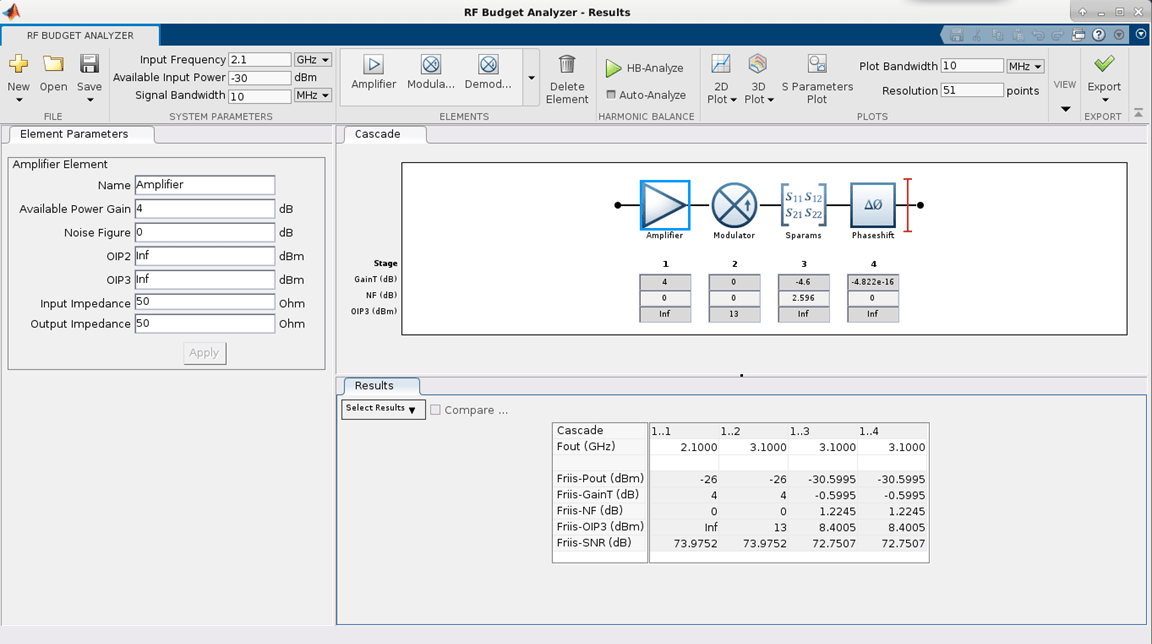# phaseshift

Create phase-shift circuit

Since R2021b

## Description

Use a `phaseshift` object to create a phase-shift circuit. The `phaseshift` object is a two-port network commonly used in image rejection receivers and digital beam steering applications.

You can also use the `phaseshift` object to model a phase shift circuit in cascaded stages using an `rfbudget` object or the RF Budget Analyzer app.

## Creation

### Syntax

``psh = phaseshift``
``psh = phaseshift(Name=Value)``

### Description

example

````psh = phaseshift` creates a `phaseshift` object with default values. ```

example

````psh = phaseshift(Name=Value)` sets Properties of the `phaseshift` object using one or more name-value arguments. For example, `psh = phaseshift(phaseshift=80)` creates an 80 degree phase shift circuit. Properties you do not specify retain their default values.```

## Properties

expand all

Name of the phase shift circuit, specified as a string scalar or a character vector.

Example: `phaseshift(Name='phaseshiftObj')`

Phase angle difference between the input and the output signals expressed by the insertion phase angle, specified as a positive scalar in degrees.

Example: `phaseshift(phaseshift=80)`

Number of input and output ports of the phase shift circuit, specified as a positive scalar.

Terminals of the phase shift circuit, specified as a cell array.

## Object Functions

 `sparameters` Calculate S-parameters for RF data, network, circuit, and matching network objects

## Examples

collapse all

` psh = phaseshift(PhaseShift = 25);`

Calculate the S-parameters at 3 GHz.

` spar = sparameters(psh,3e9)`
```spar = sparameters: S-parameters object NumPorts: 2 Frequencies: 3.0000e+09 Parameters: [2x2 double] Impedance: 50 rfparam(obj,i,j) returns S-parameter Sij ```

Create an amplifier with a gain of 4 dB.

`a = amplifier(Gain = 4);`

Create a modulator with an OIP3 value of 13 dBm.

`m = modulator(OIP3 = 13);`

Create an n-port element using `passive.s2p`.

`n = nport('passive.s2p');`

Create a 40 degree phase shift element.

`psh = phaseshift(PhaseShift = 40); `

Calculate the RF budget of a series of RF elements at an input frequency of 2.1 GHz, an available input power of –30 dBm, and a bandwidth of 10 MHz.

`b = rfbudget([a m n psh],2.1e9,-30,10e6);`

Use the `show` command at the command line to visualize the RF budget chain in the RF Budget Analyzer app. You can also do further analysis on this chain using the app. For more information, see RF Budget Analyzer.

```show(b) ```## Version History

Introduced in R2021b